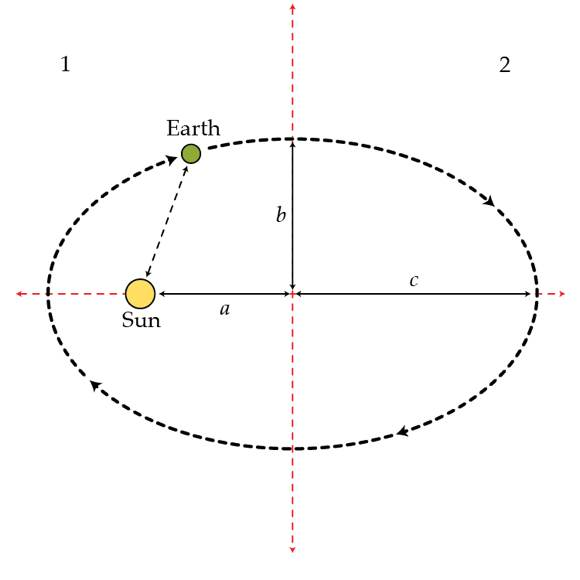# Closer to the sun

Suppose the Earth's orbit is divided in two by its minor axis as shown in the figure. How much longer in days does the Earth spend in one half than the other? The eccentricity of an elliptical orbit is defined as $e:=\frac{a}{c}$ where $c$ is the semi-major axis and $a$ is the distance from the the center of ellipse to a focal point. For the Earth's orbit this number is $e=0.0167.$×